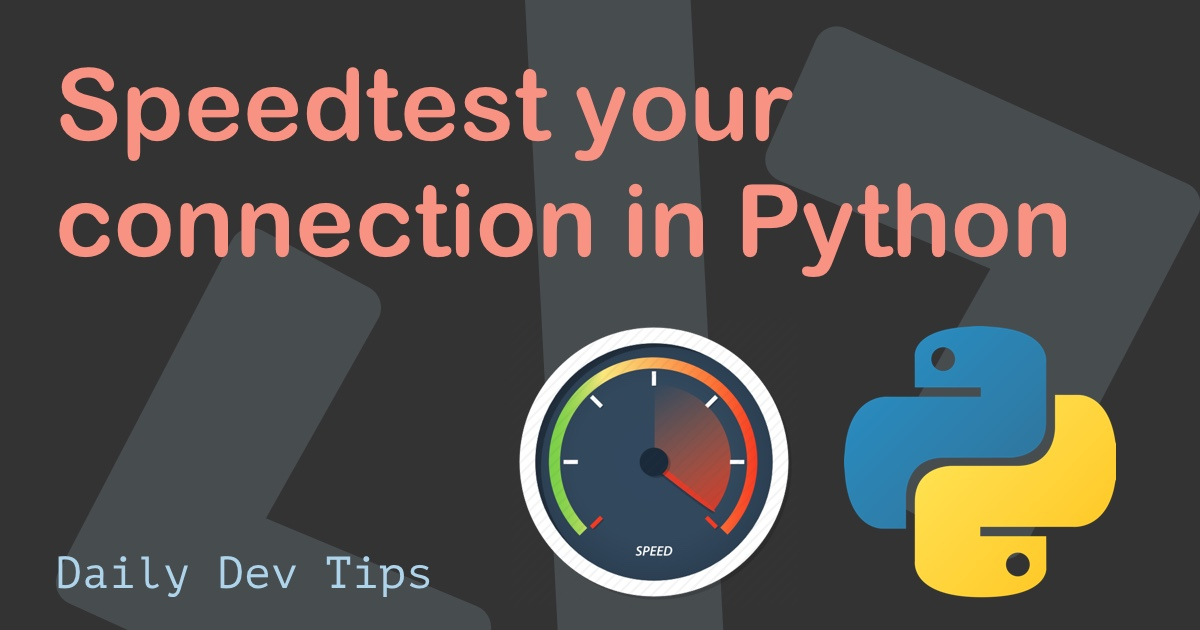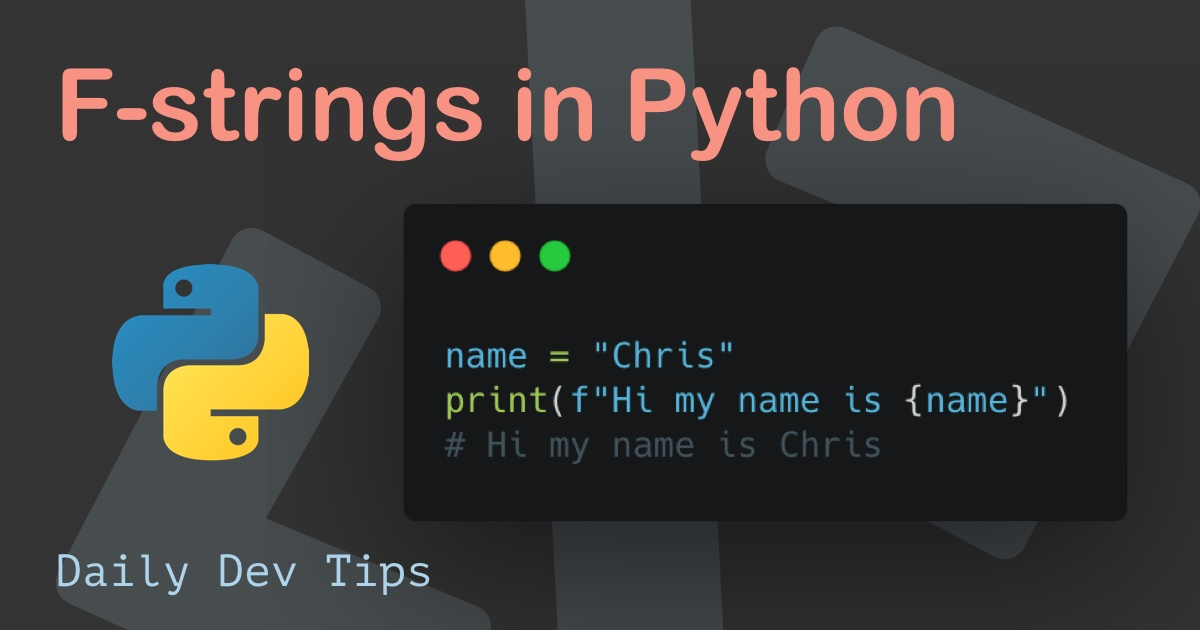Subscribe

# Writing functions in Python

••••••• + 2 devs liked it

✍️

Learn how to write functions in Python

26 May, 2021 · 2 min read

Functions are an essential part of programming, as they can execute a block of code at once. Often it's an excellent way to re-use blocks of code.

Let's give it a go and see how they work in Python.

## Creating a function in Python permalink

A function is made by prefixing the `def` keyword.

``````def foo():
print("Bar")``````

However, running our code now will not do anything since we didn't call our function yet.

To run/execute the function, we must call it somewhere in our code. In general, this happens when a specific criterion is matched, but let's just run it as is.

``````def foo():
print("Bar")

foo()``````

When we run our code now, it returns `Bar`

## Passing and returning data permalink

Having a function that prints out something is not convenient so let's see how we can give it data and return something.

Let's say we want to make a function that multiplies a number by itself and returns the output.

Meaning, if we put in the number 5, it should run `5x5` and return `25`.

``````def multiply(number):
return number * number

print(multiply(5))``````

Running this code will indeed return `25`.

We can easily have the function accept multiple arguments like so:

``````def multiply(number, multiplier):
return number * multiplier

print(multiply(5, 10))``````

This will return `50`.

And one cool thing we can do is send the arguments based on their key value like this.

``multiply(number=5, multiplier=2)``

You might not know how many arguments you're expecting in some cases, and you can prepend the argument with an asterisk (*).

``````def feed_animals(*animals):
print(animals)
print(animals)

feed_animals('Cow', 'Chicken', 'Goat')``````

Which will return:

``````('Cow', 'Chicken', 'Goat')
Goat``````

There are some more additions to functions, but this covers the basics for Python functions.

Tweet this tip
••••••• + 2 devs liked it

### Speedtest your connection in Python

21 Jun, 2021 · 2 min read### F-strings in Python

7 Jun, 2021 · 2 min readJoin 2101 devs and subscribe to my newsletter

• 1000 articles written
• 2101 devs subscribed
• 529116 words written